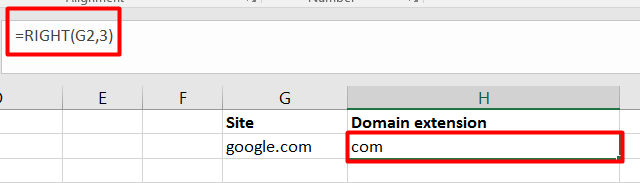# RIGHT and LEFT Functions in Excel

## Left & Right function in Excel

1. LEFT:
Definition: LEFT function in Excel returns the left-hand side characters from a text string based on the number of characters specified by the user.
Arguments/Syntax:

`LEFT(text, [num_chars])`

text: It is a required argument. It is the text string that contains the characters user wants to extract
a num_chars: It is an optional argument. It specifies the number of characters the user wants to extract from the left side. By default, it takes the value 1
Example:
a) Get the first name from the given full name:
Suppose, in excel the cell A2 contains a full name of a person as “Roland Watson”. Now, if the user wants to extract the first name then LEFT function can be used: LEFT(”Roland Watson”, 6). The output of this function is “Roland”.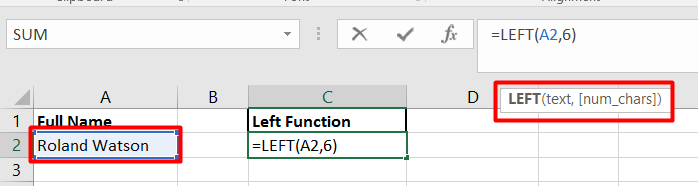b) Get the area code of given phone number:
Consider that you have a phone number like 313-512-4217 and the requirement is to extract the area code of that phone number. Now, as we know areas code is represented by the first 3 digits of the number, therefore, using the LEFT function in excel we can extract the area code: LEFT (“313-512-4217”, 3) = 313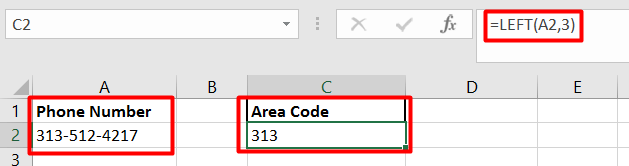c) First 3 letters of the Month
Let’s consider you have the list of all the months with their complete full name. Now, the requirement is to create the list of months name with only first 3 letters. This also can be easily achievable using LEFT function i.e. LEFT(“January”,3) = Jan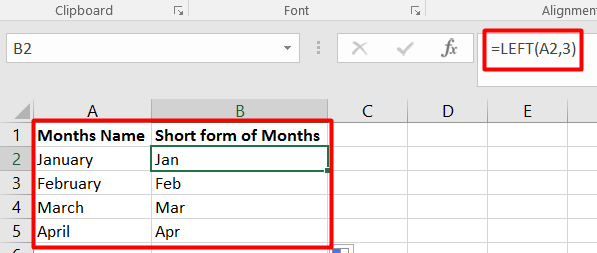2. RIGHT:
Definition: RIGHT function in excel returns the right-hand side characters from a text string based on the number of characters specified by the user.
Arguments/Syntax:

`RIGHT(text, [num_chars])`

text: It is a required argument. It is the text string that contains the characters user wants to extract
num_chars: It is an optional argument. It specifies the number of characters a user wants to extract from the right side. By default, it takes the value 1.
Example:
a) Get the last name from the given full name:
Suppose, in excel the cell A2 contains a full name of a person as “Roland Watson”. Now, if the user wants to extract the last name then the RIGHT function can be used: RIGHT(”Roland Watson” ,6). The output of this function is “Watson”.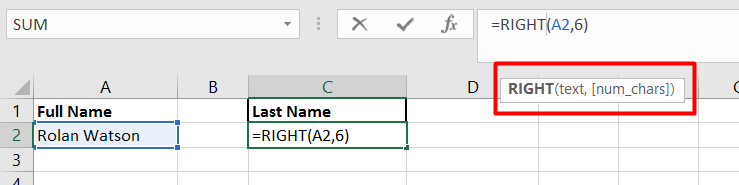b) To get the “State” abbreviation from “City, State” name:
Consider an example where we have a list of “City, State” names like “Moab, UT” and the requirement is to extract the State abbreviation from the given list in that RIGHT function can be used: RIGHT( “Moab, UT”, 2) = UT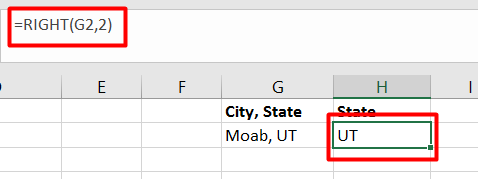c) Get the phone number without the area code:
Consider that you have a phone number like 313-512-4217 and the requirement is to extract the phone number without the area code. Now, as we know areas code is represented by the first 3 digits of the number, therefore, using the RIGHT function in excel we can extract the other part of the number: RIGHT (“313-512-4217”, 8) = 512-4217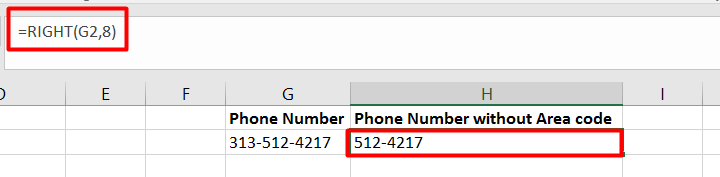d) Extracting a 3 letter domain extension:
Consider the requirement of extracting the domain extension from the given list of different types of sites. To achieve that user can use the RIGHT function in Excel: RIGHT(“google.com”,3) = com